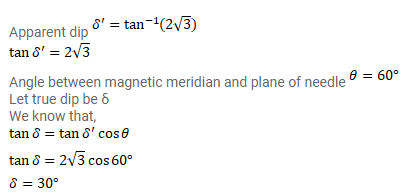# A magnetic needle is free to rotate in a vertical plane

Question:

A magnetic needle is free to rotate in a vertical plane which makes an angle of $60^{\circ}$ with the magnetic meridian. If the needle stays in the direction making an angle of $\tan ^{-1}(2 / \sqrt{3})$ with the horizontal, what would be the dip at that place?

Solution: